# Transmission Line

Delay-based or lumped parameter transmission line

•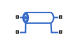Libraries:
Simscape / Electrical / Passive / Lines

## Description

The Transmission Line block lets you choose between the following models of a transmission line:

1. Delay-based and lossless

2. Delay-based and lossy

3. Lumped parameter L-section

4. Lumped parameter pi-section

5. Distributed parameter line

The first option provides the best simulation performance, with options 2, 3 and 4 requiring progressively more computing power.

### Delay-Based and Lossless

This first option, `Delay-based and lossless`, models the transmission line as a fixed impedance, irrespective of frequency, plus a delay term. The defining equations are:

 v1( t ) – i1( t ) Z0 = v2( t – τ ) + i( t – τ ) Z0 (1)

2

 v2( t ) – i2( t ) Z0 = v1( t – τ ) + i1( t – τ ) Z0 (2)

where:

• v1 is the voltage across the left-hand end of the transmission line.

• i1 is the current into the left-hand end of the transmission line.

• v2 is the voltage across the right-hand end of the transmission line.

• i2 is the current into the right-hand end of the transmission line.

• τ is the transmission line delay.

• Z0 is the line characteristic impedance.

### Delay-Based and Lossy

To introduce losses, the second option, ```Delay-based and lossy```, connects N delay-based components, each defined by the above equations, in series via a set of resistors, as shown in the following illustration.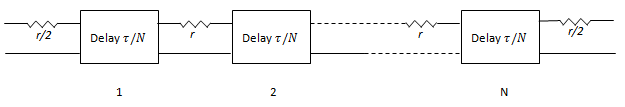N is an integer greater than or equal to 1. r = R · LEN / N, where R is the line resistance per unit length and LEN is the line length.

### Lumped Parameter L-Section

The following block diagram shows the model of one L-line segment.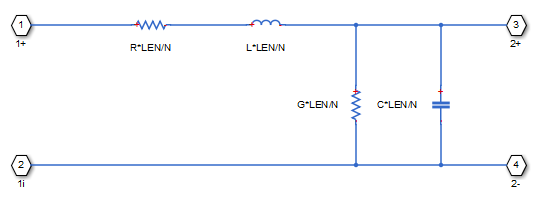The lumped parameter parameterization uses N copies of the above segment model connected in series.

Parameters are as follows:

• R is line resistance per unit length.

• L is the line inductance per unit length.

• C is the line capacitance per unit length.

• G is the line conductance per unit length.

• LEN is the length of the line.

• N is the number of series segments.

### Lumped Parameter Pi-Section

The following block diagram shows the model of one pi-line segment.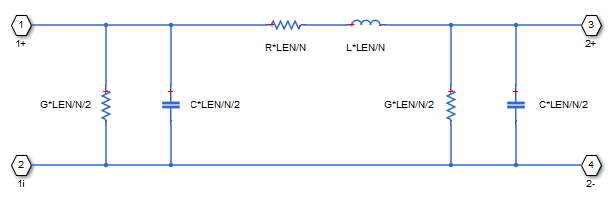The lumped parameter parameterization uses N copies of the above segment model connected in series. The parameters are as defined for the L-section transmission line model. Unlike the L-section model, the pi-section model is symmetric.

### Lumped Parameter Line Model Parameterization

The lumped-parameter models (L-section or pi-section) are the most challenging to simulate, typically needing many more segments (greater N) than for the delay-based and lossy model .

Cable manufacturers do not typically quote an inductance value per unit length, but instead give the characteristic impedance. The inductance, capacitance, and characteristic impedance are related by:

 L = C · Z02 (3)

The block lets you specify either L or Z0 when using the lumped parameter model.

### Distributed parameter line

A distributed parameter line gives more accurate simulation at particular frequency compared to the other options provided by this block. However, this is not a frequency-dependent model. The accuracy of the model will drop outside the frequency point specified in the Frequency used for rlcg specification parameter.

For a frequency-dependent transmission line model, see Frequency-Dependent Overhead Line (Three-Phase).

The electromagnetic behavior of a multiconductor transmission line is described by the telegrapher's equation.

 ${I}_{2}-{Y}_{c}{V}_{2}=-H\left({I}_{1}+{Y}_{c}{V}_{1}\right)$ (4)

 ${I}_{1}-{Y}_{c}{V}_{1}=-H\left({I}_{2}+{Y}_{c}{V}_{2}\right)$ (5)

Define:

• ${I}_{sh,1}={Y}_{c}{V}_{1}$ — Shunt current vector produced at terminal 1 by injected voltages V1

• ${I}_{sh,2}={Y}_{c}{V}_{2}$ — Shunt current vector produced at terminal 2 by injected voltages V2

• ${I}_{rfl,1}=\frac{1}{2}\left({I}_{1}+{Y}_{c}{V}_{1}\right)$ — Reflected currents of terminal 1

• ${I}_{rfl,2}=\frac{1}{2}\left({I}_{2}+{Y}_{c}{V}_{2}\right)$ — Reflected currents of terminal 2

You can then rewrite and solve equations 4 and 5:

`${I}_{1}={I}_{sh,1}-2H{I}_{rfl,2}$`

`${I}_{2}={I}_{sh,2}-2H{I}_{rfl,1}$`

The following block diagram shows the equivalent circuit for a distributed parameter line.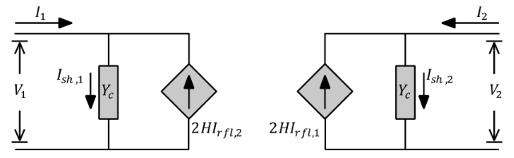## Assumptions and Limitations

• For the lumped parameter options, MathWorks recommends that you use a trapezoidal solver such as `ode23t`. This is because lumped parameter transmission models have very lightly damped internal dynamics, which are best suited to trapezoidal solvers for numerical accuracy.

• The lumped parameter pi-section model has a parallel capacitor at both ends. This means that you should not connect it directly to an ideal voltage source, that is, a source with no internal resistance. The lumped parameter L-section model, however, has a series input resistor, and therefore you can connect it directly to an ideal voltage source.

## Ports

Refer to the figure for port locations.### Conserving

expand all

Electrical conserving port associated with one end of the transmission line inner conductor.

Electrical conserving port associated with one end of the transmission line external shielding conductor.

Electrical conserving port associated with one end of the transmission line inner conductor.

Electrical conserving port associated with one end of the transmission line external shielding conductor.

## Parameters

expand all

Select one of the following transmission line models:

• `Delay-based and lossless` — Model the transmission line as a fixed impedance, irrespective of frequency, plus a delay term, as described in Delay-Based and Lossless. This is the default method. It provides the best simulation performance.

• `Delay-based and lossy` — Model the transmission line as a number of delay-based components, connected in series via a set of resistors, as described in Delay-Based and Lossy.

• `Lumped parameter L-section` — Model the transmission line as a number of L-line segments, connected in series, as described in Lumped Parameter L-Section.

• `Lumped parameter pi-section` — Model the transmission line as a number of pi-line segments, connected in series, as described in Lumped Parameter Pi-Section.

• `Distributed parameter line` — Model the transmission line as a distributed parameter line, as described in Distributed parameter line.

The total transmission line delay. The parameter value must be greater than zero. The default value is `5` ns, which is a typical value for a one-meter coaxial cable.

#### Dependencies

This parameter is visible only when you select `Delay-based and lossless` or `Delay-based and lossy` for the Model type parameter.

Select the model parameterization method, as described in Lumped Parameter Line Model Parameterization:

• ```By characteristic impedance and capacitance``` — Specify values for the Characteristic impedance and Capacitance per unit length parameters. This is the default method.

• `By inductance and capacitance` — Specify values for the Inductance per unit length and Capacitance per unit length parameters.

#### Dependencies

This parameter is visible only when you select ```Lumped parameter L-section``` or ```Lumped parameter pi-section``` for the Model type parameter.

The characteristic impedance of the transmission line. The parameter value must be greater than zero.

#### Dependencies

This parameter is visible only when you select either:

• `Delay-based and lossless` or `Delay-based and lossy` for the Model type parameter.

• `Lumped parameter L-section` or `Lumped parameter pi-section` for the Model type parameter and ```By characteristic impedance and capacitance``` for the Parameterization parameter.

Frequency used for the R, L, C, G specification, where:

• R is line resistance per unit length.

• L is the line inductance per unit length.

• C is the line capacitance per unit length.

• G is the line conductance per unit length.

#### Dependencies

This parameter is visible only when you select ```Lumped parameter L-section```, ```Lumped parameter pi-section```, or ```Distributed parameter line``` for the Model type parameter.

The effective inductance of the transmission line per unit length. The parameter value must be greater than zero.

#### Dependencies

This parameter is visible only when you select ```Lumped parameter L-section```, ```Lumped parameter pi-section``` or ```Distributed parameter line``` for the Model type parameter and `By inductance and capacitance` for the Parameterization parameter.

The transmission line capacitance per unit length.

#### Dependencies

This parameter is visible only when you select ```Lumped parameter L-section```, ```Lumped parameter pi-section``` or ```Distributed parameter line``` for the Model type parameter.

The total transmission line resistance (that is, the sum of the resistance for the two conducting paths) per unit length.

#### Dependencies

This parameter is visible only when you select `Delay-based and lossy`, `Lumped parameter L-section`, `Lumped parameter pi-section` or `Distributed parameter line` for the Model type parameter.

The conductance between the two transmission line conductors per unit length. The parameter value must be greater than, or equal to, zero.

#### Dependencies

This parameter is visible only when you select ```Lumped parameter L-section```, ```Lumped parameter pi-section``` or ```Distributed parameter line``` for the Model type parameter.

The total transmission line length. The parameter value must be greater than zero.

#### Dependencies

This parameter is visible only when you select `Delay-based and lossy`, `Lumped parameter L-section`, `Lumped parameter pi-section` or `Distributed parameter line` for the Model type parameter.

The number of model segments used to represent the transmission line. The parameter value must be an integer greater than, or equal to, 1.

#### Dependencies

This parameter is visible only when you select `Delay-based and lossy`, `Lumped parameter L-section` or `Lumped parameter pi-section` for the Model type parameter.

 Sussman-Fort, S.E. and J.C. Hantgan. “SPICE Implementation of Lossy Transmission Line and Schottky Diode Models.” IEEE Transactions on Microwave Theory and Techniques. Vol. 36, No. 1, January, 1988.